# Relativity of measures

• B
AlMetis
A frame “A” of three perpendicular axis (x, y, z) and an identical frame “B”, with an observer at rest in each, are moving toward each other at 1/2c on their common x axis.
Centered between A and B on x is a light source S.
At the time t1 when A, B and S coincide on x, S emits a light flash.
Given the symmetry of motion between A, B and S, are the paths observed by A and B a mirror of each other?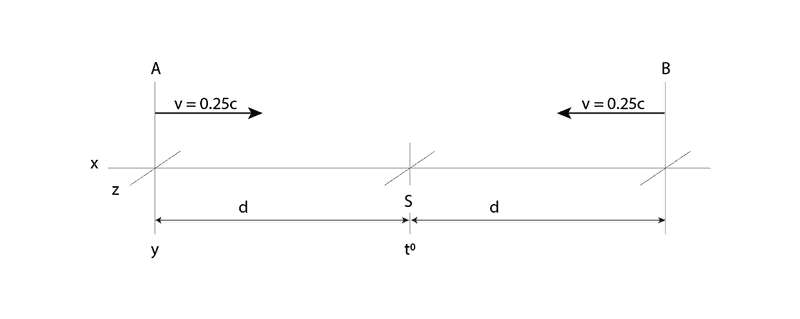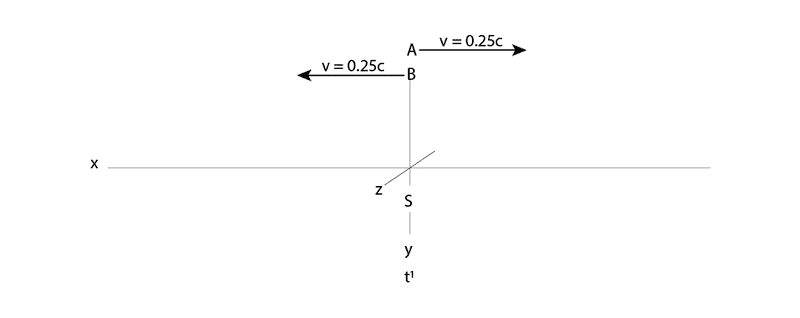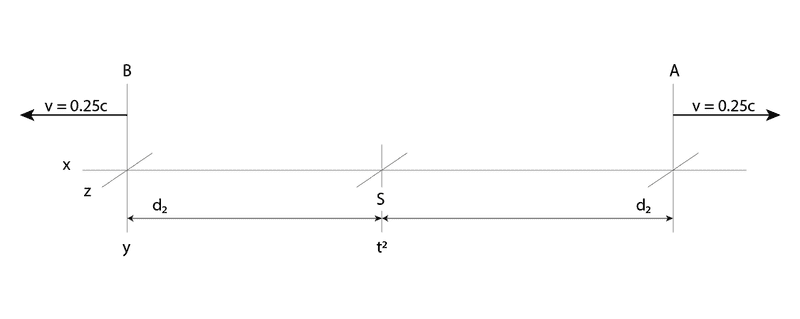2022 Award
A frame “A” of three perpendicular axis (x, y, z) and an identical frame “B”, with an observer at rest in each,
That's not really what's meant by a frame, but that doesn't really matter here.
moving toward each other at 1/2c on their common x axis.
This is more problematic. Your diagram implies both are doing 0.25c in the frame where their speeds are equal and opposite, but this does not mean either is doing 0.5c with respect to any object in your scenario.
Given the symmetry of motion between A, B and S, are the paths observed by A and B a mirror of each other?
What do you mean by "paths"? Paths of what?

And since this looks like homework, why don't you give your answer and justification first?

Homework Helper
Gold Member
The answer depends on what you assume and what you want to conclude. You say nothing about whether these are inertial reference frames or not. For all we know, the entire set-up might be whizzing around, accelerating, and spinning. Mathematical reference frames can do that.
I assume that you meant them to be inertial reference frames. Then yes, there is symmetry. Otherwise, there is not necessarily physical symmetry. With acceleration, the behavior of the light could be different (not symmetric) for observers A and B.

AlMetis
I think this means the speed between A and B is 0.5c, because they are both moving toward S with speed 0.25c

I think this is true. A photon going straight up from S would appear to angle toward B and vise a versa because A and B are going in opposite directions.

2022 Award
I think this means the speed between A and B is 0.5c, because they are both moving toward S with speed 0.25c
Then you need to look up relativistic velocity addition. Each one sees the other doing 8c/17, or about 0.47c.
I think this is true. A photon going straight up from S would appear to angle toward B and vise a versa because A and B are going in opposite directions.
Which observer would see this?

Also, a word to the wise, don't talk about photons in relativity. Talk about light pulses. Photons are much more complicated things and it's good not to refer to classical light pulses as photons. Then you will end up less confused when you study actual photons in quantum field theory.

•hutchphd and topsquark
AlMetis
Is it more accurate to say a light pulse perpendicular to x as seen from S, would appear at an angle toward B when seen from A and the same angle toward A when seen from B?

AlMetis
FactChecker, yes these are inertial frames of reference.

2022 Award
Assuming A and B have passed the source and are moving away from it, yes.

AlMetis
From the inertial frame “E”, also on the common x axis with A, B and S, an observer measures the motion of S at 0.125 c along x toward B, the motion of A at 0.375c along x toward B, and the motion of B at 0.125 c along x toward S.
At the time t1 A, B, S and E coincide on x.
E remains at the center of the light pulse while A, B and S continue in their motion relative to E.
Given the asymmetry of the motion between A, B and S relative to E, at t1, would a light pulse viewed from E moving perpendicular to x, appear at an angled toward B when seen from A and the same angle toward A when seen from B?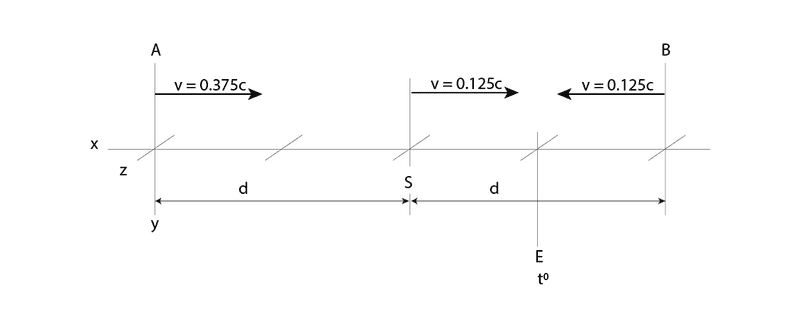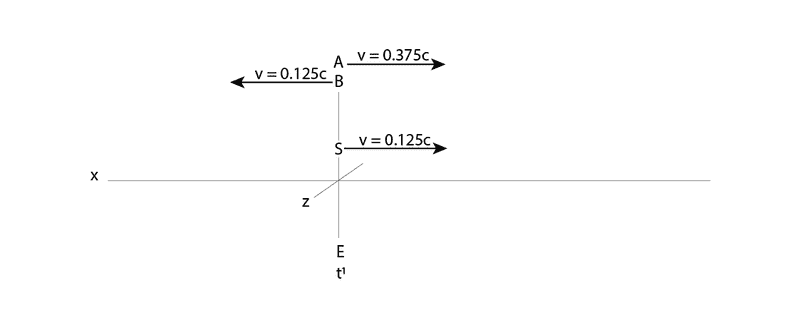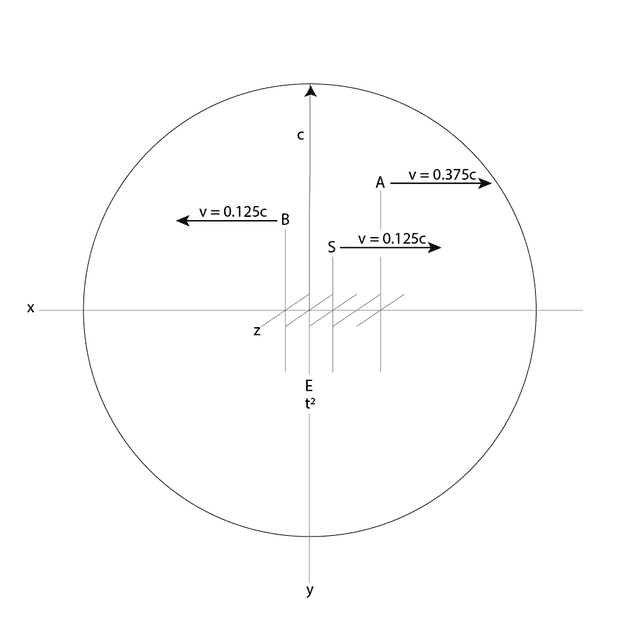Mentor
Assuming that all the speeds are stated relative to the origin of the same inertial frame (so no need to mess with relativistic velocity addition)….
Assuming that we’re considering the light source is something like a laser, sending a flash out on a straight-line path (as opposed to the expanding sphere of light we’d get by switching on a light bulb)….
Assuming that in that frame the laser was aimed straight up, perpendicular to the x-axis….

A will find that the path of the laser light is not perpendicular to the x-axis. Instead it is at some non-zero angle ##\theta## from the perpendicular. (I’m not going to bother calculating it because the exact numerical value is uninteresting, the point is that it is non-zero).
B will find that the path of the laser light is not perpendicular to the x-axis. It will be at an angle ##-\theta## to the perpendicular. This is clear from the symmetry of the setup and is just a repeat of the discussion up through post #8.
E will find that the path of the laser light is straight up, perpendicular to the x-axis. That’s part of the specification of the problem, it’s the third assumption above.

All three agree about where the light beam is actually going. The difference in their descriptions of the path is because they have different notions of ”perpendicular”. It’s similar to the trajectory of a bouncing ball on the deck of a ship - vertical relative to the deck if we’re on the ship, angled relative to the deck if we’re on the shore watching the ship moving past us.

Your setup you is one mirror away from being a light clock that is at rest relative to E, so you may want to Google for “light clock” and look at some of our older threads on the subject.

•hutchphd
AlMetis
A light clock does make this a clearer picture, thank you.

It appears in the first example A and B would see what you said ##\theta## and ##-\theta## which I assume is a mirror on x across y.
But the second example puts A further along +x than B is along -x.
Why doesn’t this mean A sees a different angle that what B sees?

Mentor
But the second example puts A further along +x than B is along -x.
Why doesn’t this mean A sees a different angle that what B sees?
I'm sorry, I wasn't looking carefully and didn't notice that you had selected different speeds in the second example.

2022 Award
From the inertial frame “E”, also on the common x axis with A, B and S, an observer measures the motion of S at 0.125 c along x toward B, the motion of A at 0.375c along x toward B, and the motion of B at 0.125 c along x toward S.
These numbers are inconsistent with your initial setup. You appear to have used Galilean velocity transformation to E's rest frame, not relativistic.

Assuming that the intent is that E and S measure each other to be doing ##c/8## then E would measure A to be doing ##4c/11## and B to be doing ##4c/31##. On the other hand, if the intent is that E measure S and B to be doing the same speed then it would need to be doing ##(4-\sqrt{15})c## relative to S and would measure A to be doing ##(4\sqrt{15}-17)c/(\sqrt{15}-8)##.

Last edited:
•malawi_glenn
AlMetis
The second example is not a relativistic transformation of the first, each is the same motion defined in Galilean measures relative to S in the first example and relative to E in the second example.

Whether the speeds are Galilean, or relativistic measures (because the observations of A and B are either symmetric, or not), the light pulse observed from A and B are different in each example?

2022 Award
The second example is not a relativistic transformation of the first, each is the same motion defined in Galilean measures relative to S in the first example and relative to E in the second example.
Ok. Probably worth stating when you are starting a new example, especially if you reuse numbers and labels. It seems to have caught out both Nugatory and me for different reasons.
Whether the speeds are Galilean, or relativistic measures (because the observations of A and B are either symmetric, or not), the light pulse observed from A and B are different in each example?
They would be symmetric in the first (A, B, S) example and not in the second (A, B, S, E).

Where are you going with all this? It's fairly straightforward to do the Lorentz transforms and determine all of this.

AlMetis
Sorry for the confusion.

I’m asking why they are different.

How is the symmetry of the observations of a light pulse by two inertial frames (A and B) broken simply by the knowledge of the motion relative to another inertial frame E?

2022 Award
How is the symmetry of the observations of a light pulse by two inertial frames (A and B) broken simply by the knowledge of the motion relative to another inertial frame E?
It isn't. In one case you emitted a light pulse perpendicular to the x axis as seen by S, who sees A and B moving with equal speed. In the second case it was emitted perpendicular to the x axis as seen by E, who sees A moving faster than B. So this version of the scenario does not have mirror symmetry in any frame. The first one does. You broke the symmetry by specifying an asymmetric scenario.

Last edited:
AlMetis
Yes I did, I am confusing the angle of emission with the path observerd.

In each example the light pulse is emitted perpendicular to x, which is to say the direction, or pointing of the source is perpendicular to x and x is common to all observers. The angle of the path traced by the light pulse between t1 and t2 is determined by the motion of the observer relative to the pulse.

I think what is confusing is that the first example does not give enough information to know the path observed by A and B. The second example shows A, B and S are moving, but without the inertial frame E, we don’t know they are.

Homework Helper
Gold Member
2022 Award
I think what is confusing is that the first example does not give enough information to know the path observed by A and B. The second example shows A, B and S are moving, but without the inertial frame E, we don’t know they are.
None of this makes any sense to me, I'm sorry to say. I'm struggling to see the purpose of this thread?

2022 Award
I think what is confusing is that the first example does not give enough information to know the path observed by A and B.
Yes it does, at least as modified by #6 where you clarified that the light pulse is emitted perpendicular to the frame velocities as seen by S.
The second example shows A, B and S are moving, but without the inertial frame E, we don’t know they are.
No, you can always pick one frame and choose to call it a rest frame. You chose E there, but you could have chosen any of the other three, or some other frame that doesn't have a physical manifestation in your scenario. The difference between your scenarios is entirely due to your differing choices of setup; in both scenarios at most one of the frames can be chosen to be at rest and all the others are moving. No analysis can possibly show otherwise.

AlMetis
I understand that the position of rest is relative, but the choice of an observer at one location should not change what is observed by another observer at another location.

I will use your suggestion of a light clock.
When the emitter at the bottom of the clock is pointing at the mirror at the top of the clock, the light pulse will NOT strike the mirror if the mirror moves after emission. We are talking about a pulse that travels in a straight line in the direction the emitter is pointed.
Whether the pulse strikes the mirror or not is not something determined by an observer’s choice of reference frame, even though whether the mirror moves or not is “exclusively” determined by the observer’s choice of reference frame. So an observer can choose a frame that explains the empirical evidence, and they can choose a frame that contradicts the empirical evidence.
We could say the light pulse scenario is just a tool for discussion and the reality is light is a wave so the light will strike the mirror even when the mirror moves after emission. That makes more sense, but the light pulse example makes it clear that even using the wave model we have to admit the distance from emitter to mirror is greater when the mirror moves after emission.

•PeroK
I will use your suggestion of a light clock.
When the emitter at the bottom of the clock is pointing at the mirror at the top of the clock, the light pulse will NOT strike the mirror if the mirror moves after emission.
That's wrong. If the angle of the light pulse trajectory with the x-axis is 90 degree in the rest frame of the light-clock, then it is not 90 degree in the other frame of reference. This difference of the angle in two frames is called "aberration".

•hutchphd, malawi_glenn, vanhees71 and 1 other person
2022 Award
the choice of an observer at one location should not change what is observed by another observer at another location.
Indeed. Nobody has said otherwise and a physical model that predicted that would be rapidly discarded.
When the emitter at the bottom of the clock is pointing at the mirror at the top of the clock, the light pulse will NOT strike the mirror if the mirror moves after emission.
If you mean a light clock that accelerates perpendicular to its length then I agree, and all observers will agree. If you mean a light clock viewed from a frame where it is in steady motion then no, the light pulse will always be found on a line between emitter and mirror and all frames will agree on this.
We are talking about a pulse that travels in a straight line in the direction the emitter is pointed.
No - as @Sagittarius A-Star comments, you appear not to understand aberration. It's extremely common for motions that are perpendicular in one frame not to be perpendicular in others. The most prosaic is rain on a car window. The car moves horizontally and the rain falls vertically, yet the rain makes slanted drops on the side windows of the car. This shows that, in the rest frame of the car, the rain isnot falling vertically.
Whether the pulse strikes the mirror or not is not something determined by an observer’s choice of reference frame,
True.
So an observer [...] can choose a frame that contradicts the empirical evidence.
No they cannot.
We could say the light pulse scenario is just a tool for discussion and the reality is light is a wave so the light will strike the mirror even when the mirror moves after emission. That makes more sense, but the light pulse example makes it clear that even using the wave model we have to admit the distance from emitter to mirror is greater when the mirror moves after emission.
This seems to be you looking for a way out of a trap you built for yourself by not following the point about aberration.

Homework Helper
Gold Member
2022 Award
When the emitter at the bottom of the clock is pointing at the mirror at the top of the clock, the light pulse will NOT strike the mirror if the mirror moves after emission. We are talking about a pulse that travels in a straight line in the direction the emitter is pointed.
If that were true, it would apply to all objects, not just light ways. E.g. imagine you are in a moving car and you throw an object out of the side window in a straight line perpendicular to the side of the car (from your reference frame). To an observer on the ground, the object moves at an angle relative to the side of the car.

There is no contradiction here. Instead, it's your misconception that somehow the relative direction of motion must be the same in both frames (which is physically impossible).

There seem to be many students who make this mistake. It may boil down to it being difficult to imagine the same scenario in two different reference frames.

In the above example, imagine you fire the object using a length of tube pointing out the car. To an observer on the ground, the object will still move at an angle (because the tube is moving). In fact, you can consider the motion of the object as it moves down the tube. To the observer in the car, it is moving perpendicular to the side of ther car. But, to the observer on the ground, it is moving at an angle. To maintain your assertion, then the object must break out of the tube in the ground frame to move perpendicular to the car - which is physically impossible.

2022 Award
It may boil down to it being difficult to imagine the same scenario in two different reference frame.
I think it comes down to this: if I hold my arm out to the side and walk forward my arm is perpendicular to my direction of motion and/or the direction of motion of the floor in all frames. But if an ant walks down my arm, it is not moving perpendicular to my/the floor's motion in most frames, despite walking on my perpendicular arm.

That may seem paradoxical, but if so then you (general you, not @PeroK) are mixing up your frames of reference and forgetting to keep track of what is moving in which frame.

•vanhees71, jbriggs444 and PeroK
Homework Helper
Gold Member
2022 Award
I think it comes down to this: if I hold my arm out to the side and walk forward my arm is perpendicular to my direction of motion and/or the direction of motion of the floor in all frames. But if an ant walks down my arm, it is not moving perpendicular to my/the floor's motion in most frames, despite walking on my perpendicular arm.

That may seem paradoxical, but if so then you (general you, not @PeroK) are mixing up your frames of reference and forgetting to keep track of what is moving in which frame.
That's the perfect example!

•Ibix
Gold Member
•••hutchphd, Dale, Sagittarius A-Star and 4 others
Gold Member
2022 Award
That's wrong. If the angle of the light pulse trajectory with the x-axis is 90 degree in the rest frame of the light-clock, then it is not 90 degree in the other frame of reference. This difference of the angle in two frames is called "aberration".
Aberration is explained in Sect. 4.3 on the Doppler effect of light in

https://itp.uni-frankfurt.de/~hees/pf-faq/srt.pdf

AlMetis
@PeroK, “Objects” acquire momentum from their source (cars), light does not.

As DrGreg’s animation illustrates, the equilateral triangle (source - mirror- source) is the path of the light pulse. It is not what is measured by an observer in the train(aberration), but it is the physical path the pulse must take to meet the mirror when the mirror is no longer “physically” where it was at the time of emission.

If that was not the case there would be no need to reconcile two “different” lengths measured by an observer on the platform with an observer in the train (and at very hight speeds invoke the Lorentz transformation). We would simply say, one of the observers is wrong.

When there is an identical source on the platform that emits the pulse when the source on the train aligns with it on the track (x axis), the observations/measurements would not change for either observer from those they made when the pulse was emitted from the source on the train. For all intents and purposes the observer on the train would not have any way to measure, or reason to even suspect the pulse was not emitted from the source on the train.
This demonstrates the position of the source at emission relative to the position of the mirror at reflection is what determines the length the light pulse travels from source to reflection. This length is a “measurement” not common to both observers, but it is common and a LONGER measure for all observers at rest with the source (platform) and it is common and a SHORTER measure for all observers at rest with the train.

•weirdoguy
Homework Helper
Gold Member
2022 Award
@PeroK, “Objects” acquire momentum from their source (cars), light does not.
Yes, it does. It must, otherwise (as you have discovered), the motion of light is physically completely impossible. Imagine light moving down the centre of a hollow tube (in vacuum). It must move down the centre of that hollow tube in all frames (just like any object). It can't move inside the tube in one frame and outside the tube in another frame where the tube is moving.

Like I said, a lot of students share your misconception. The simple fact is that light cannot possibly behave the way you demand, so you must have misunderstood something!

•vanhees71 and Ibix
Homework Helper
Gold Member
2022 Award
PS it's the speed of light that's invariant. Not its momentum vector.

•vanhees71, Vanadium 50, robphy and 1 other person
2022 Award
“Objects” acquire momentum from their source (cars), light does not.
Yes it does. You may be mistaking the (true) statement that the speed of light is independent of its source for the (false) one that the velocity of light is independent of its source. As PeroK has already pointed out, your (mis)conception of how light behaves is internally inconsistent, which is why you are struggling with it.

•vanhees71 and PeroK
Homework Helper
Gold Member
PS it's the speed of light that's invariant. Not its momentum vector.

Indeed, blue light has larger components for its 4-momentum vector compared to red light. But both blue light and red light travel at the same speed.

•vanhees71
Whether the pulse strikes the mirror or not is not something determined by an observer’s choice of reference frame
Exactly! Therefore, if you agree, that the light-pulse strikes the mirror in the rest-frame of the light-clock, then you must also agree, that the light-pulse strikes the mirror in the other reference-frame.

•vanhees71 and PeroK
In fact, why don't we do some maths? Let's say an object at rest at the origin emits a pulse of light in the ##+y## direction at ##t=0##. The coordinates of the light pulse at time ##t## are therefore ##x=0, y=ct##. Plug in the Lorentz transforms:$$\begin{eqnarray*} t'&=&\gamma(t-vx/c^2)\\ &=&\gamma t\\ x'&=&\gamma(x-vt)\\ &=&-\gamma vt\\ &=&-vt'\\ y'&=&y\\ &=&ct\\ &=&ct'/\gamma \end{eqnarray*}$$So we can see that relativity says that the light pulse's coordinates in a frame where the source is moving are ##x'=-vt## and ##y'=ct'/\gamma##. The speed is still ##c##: ##x'^2+y'^2=(v^2+c^2/\gamma^2)t'^2=c^2t'^2## so the pulse is distance ##ct'## from the origin at time ##t'##. But its velocity has acquired an ##x## component so is not perpendicular to the ##x## axis in this frame.
•